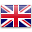# Intermediate

Sort by:
##### 1.5 時間到了! Time's Up!

STEM lessons + blocks

Students will solve word problems involving addition and...##### 1.6 房子牢固性測試 Build it up, break it down

STEM lessons + blocks

Students will investigate how a house can be...##### 1.7 種子的傳播 Seed Dispersal

STEM lessons + blocks

Students will gain understanding of seed dispersal as...##### 2.5 智能門鈴 Smart Door Bell

STEM lessons + blocks

Students will look at the use of sensors...##### 2.6 磁力 Magnetic Force

STEM lessons + blocks

Students will work collaboratively to investigate cause and...##### 2.7 打鼓模擬器 Drum Simulator

STEM lessons + blocks

Students will gain understanding of how the speed...##### 3.5 火星探測號 Mars Rover

STEM lessons + blocks

Students will identify two possible solutions for designing...##### 3.6 燈塔 Lighthouse

STEM lessons + blocks

Students will investigate how light reflecting from objects...##### 3.7 信息的傳遞 SAM Insect

STEM lessons + blocks

Students will work collaboratively to investigate how information...##### 4.4 摩斯密碼警報 Morse Code Alert

STEM lessons + blocks

Students will extend their knowledge of Morse Code,...##### 4.5 密碼與模式 Password and Patterns

STEM lessons + blocks

In the first session, students will...##### 4.6 牛頓第三運動定律 Every Action ....

STEM lessons + blocks

Students will gain understanding of Newton’s Third Law...##### 4.7 探索遺傳 Exploring Heredity

STEM lessons + blocks

Students will work collaboratively to investigate heredity and...